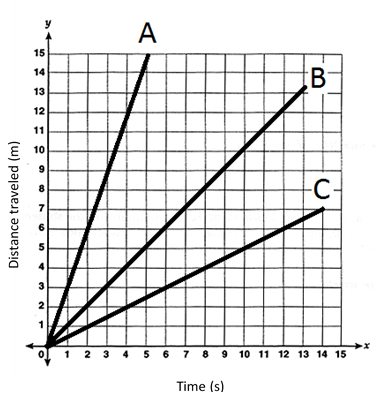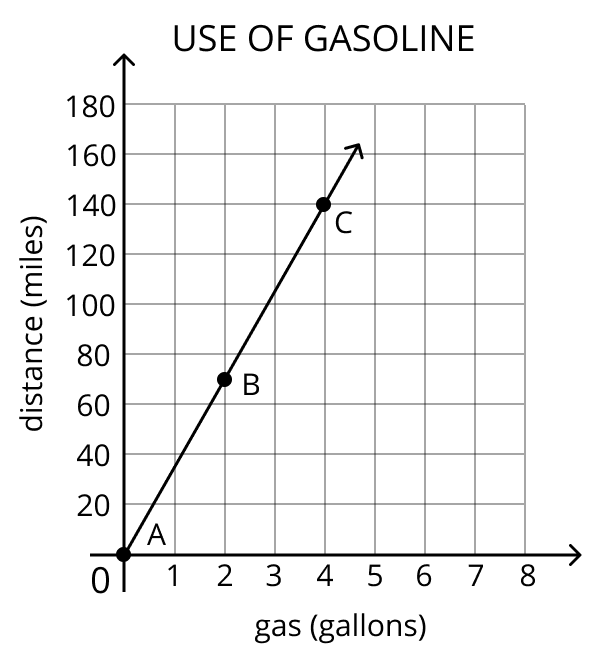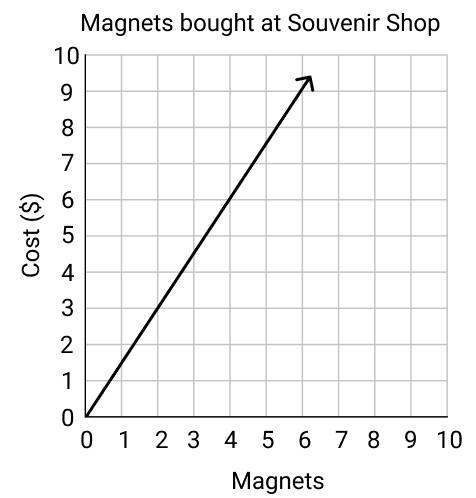# Proportional Relationships

## Objective

Interpret proportional relationships represented in graphs.

## Common Core Standards

### Core Standards

?

• 7.RP.A.2 — Recognize and represent proportional relationships between quantities.

• 7.RP.A.2.D — Explain what a point (x, y) on the graph of a proportional relationship means in terms of the situation, with special attention to the points (0, 0) and (1, r) where r is the unit rate.

?

• 6.RP.A.3.A

## Criteria for Success

?

1. Identify the constant of proportionality in a graph by finding the value of $y/x$ from coordinate point $(x,y)$
2. Identify the constant of proportionality or unit rate as the value $r$, when $x$ is $1$, represented by the coordinate point $(1,r)$
3. Explain the meaning of $(0, 0)$ in context of the situation.

## Tips for Teachers

?

• Students reason abstractly as they represent proportional situations using tables and graphs, and interpret the information to identify the constant of proportionality and write an equation. Given a graph of a proportional relationship, students re-contextualize information represented in coordinate points to explain what $(0, 0)$ and $(0, r)$ mean in context of the problem (MP.2).
• The following materials are used or would be useful in this lesson: graph paper, rulers.

#### Remote Learning Guidance

If you need to adapt or shorten this lesson for remote learning, we suggest prioritizing Anchor Problem 3 (benefits from worked example). Find more guidance on adapting our math curriculum for remote learning here.

#### Fishtank Plus

• Problem Set
• Student Handout Editor
• Vocabulary Package

## Anchor Problems

?

### Problem 1

In a video game, for every 3 coins you collect, you earn 4 points.

1. Create a table of values to represent the relationship.
2. Graph the relationship.
3. Determine the equation that represents the relationship.

### Problem 2

Three toy cars race down a straight path. The distance each car traveled in meters, over time measured in seconds, is shown in the graph below.1. At what speed is each car traveling? Where can you see each speed in the graph?
2. Explain the significance of the coordinate point $(1, r)$. What do you think the “$r$” represents?

### Problem 3

The graph below shows the number of gallons of gasoline used by a car over a given number of miles.1. Explain what each point A, B, and C mean in context of the situation.
2. Plot the point that represents the unit rate. Explain how you determined your answer, and what it means in context of the situation.
3. Does this car get more or less miles per gallon of gasoline than a car that gets 30 miles per gallon?

## Problem Set

?The following resources include problems and activities aligned to the objective of the lesson that can be used to create your own problem set.

A souvenir shop sells magnets, 2 for $3. The graph below shows the proportional relationship.Which statements below are true? For each false statement, correct it so that the statement reads true. 1. The point (6, 4) on the graph means that 6 magnets cost$4.
3. If a customer purchases 0 magnets, they will pay $0, which is represented by the point (0, 0). 4. The point (0.67, 1) means that each magnet costs$0.67.